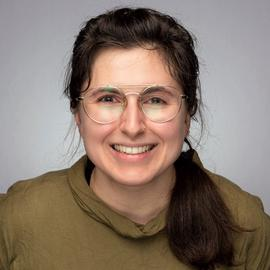Generative art using ggplot2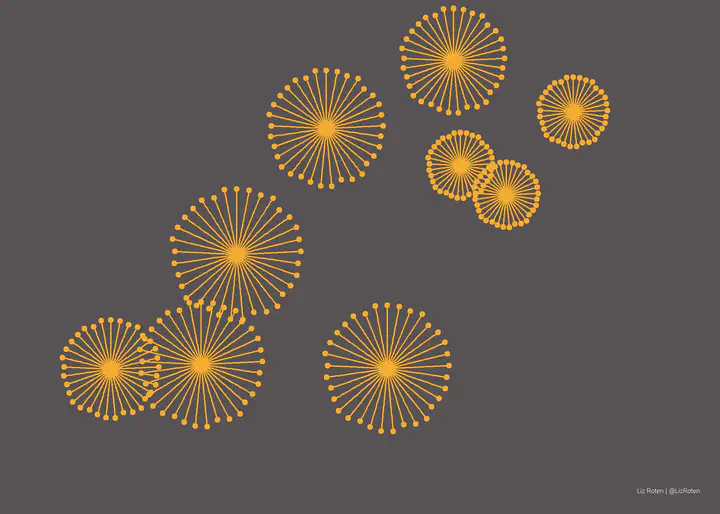I’ve been obsessed with Ijeamaka Anyene’s art for months now. Her zine is gorgeous, and I’ve been antsy to try some of the techniques out.

## Spokes: Pattern 01 Variants

# setup
library(tidyverse)
library(colorspace)
set.seed(246153)

library(showtext)
showtext_auto()
file <- sysfonts::font_files()

my_caption <- "Liz Roten | @LizRoten"


See original here.

lines <- tibble(
x = seq(0, 29, by = 0.2),
xend = rep(0,146),
y = runif(146, min = 5, max = 9),
yend = rep(0,146),
speed = 3
)

ggplot() +
geom_segment(data = lines,
aes(x = x,
y = y,
xend = xend,
yend = yend),
color = "white",
alpha = 0.5) +
geom_point(data = lines,
aes(x = x,
y = y),
color = "white") +
coord_polar() +
scale_x_continuous(limits = c(0, 29)) +
# scale_y_continuous(limits = c(-1, 10)) +
labs(caption = my_caption) +
theme_void() +
theme(plot.background = element_rect(fill = "#14342B",
color = NA),
panel.background = element_rect(fill = "#14342B",
color = NA),
plot.caption = element_text(family = "Nunito Light",
color = "white",
size = 12),
plot.margin = unit(c(.5, .5, .2, .5), "cm")
)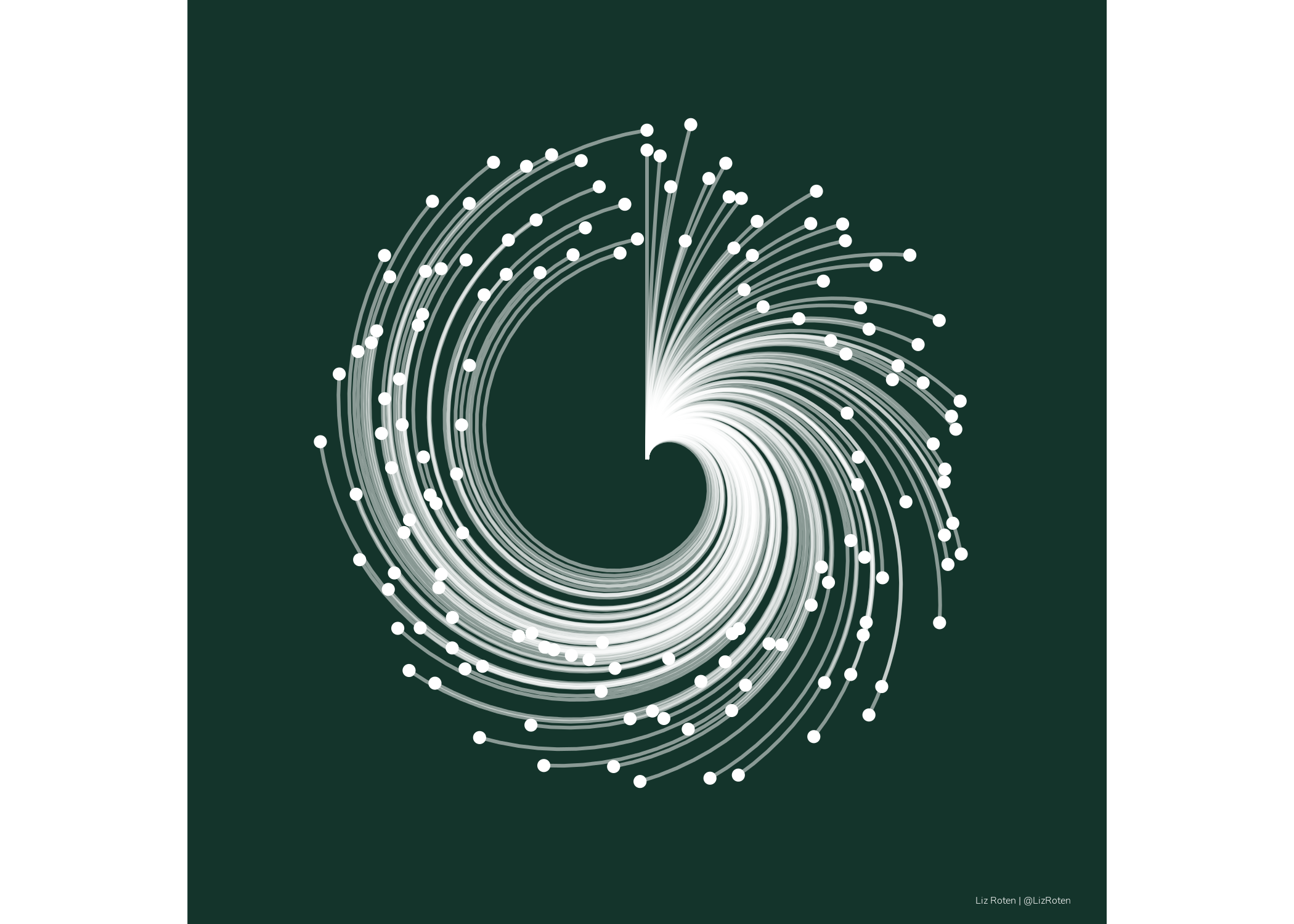## Spokes: Pattern 10 Variants

See original here.

increment = .05

circle_1 = tibble(
x = seq(from = 0, to = 5, by = increment),
xend = seq(from = 0, to = 5, by = increment),
y = 0,
yend = 1.4,
type = LETTERS)

circle_2 = tibble(
x = seq(from = 0, to = 5, by = increment  + increment),
xend = seq(from = 0, to = 5, by = increment + increment),
y = 1.5,
yend = 2.9,
type = LETTERS)

circle_3 = tibble(
x = seq(from = 0, to = 5, by = increment ),
xend = seq(from = 0, to = 5, by = increment  ),
y = 3,
yend = 4.5,
type = LETTERS)

palette_values = c("#94524A", "#8d667e", "#39355c")

bind_rows(circle_1, circle_2, circle_3) %>%
ggplot(.) +
geom_segment(aes(x = x,
y = y,
xend = xend,
yend = yend,
color = type),
show.legend = F) +
scale_color_manual(values = palette_values) +
labs(caption = my_caption) +
coord_polar() +
theme_void() +
theme(
# plot.background = element_rect(fill = "#565254",
#                                  color = NA,
#                                  size = 0),
#   panel.background = element_rect(fill = "#565254",
#                                   color = NA,
#                                   size = 0),
plot.caption = element_text(family = "Nunito Light",
color = "black",
hjust = 1,
size = 14),
plot.caption.position = "plot",
plot.margin = unit(c(.5, .5, .5, .5), "cm")
)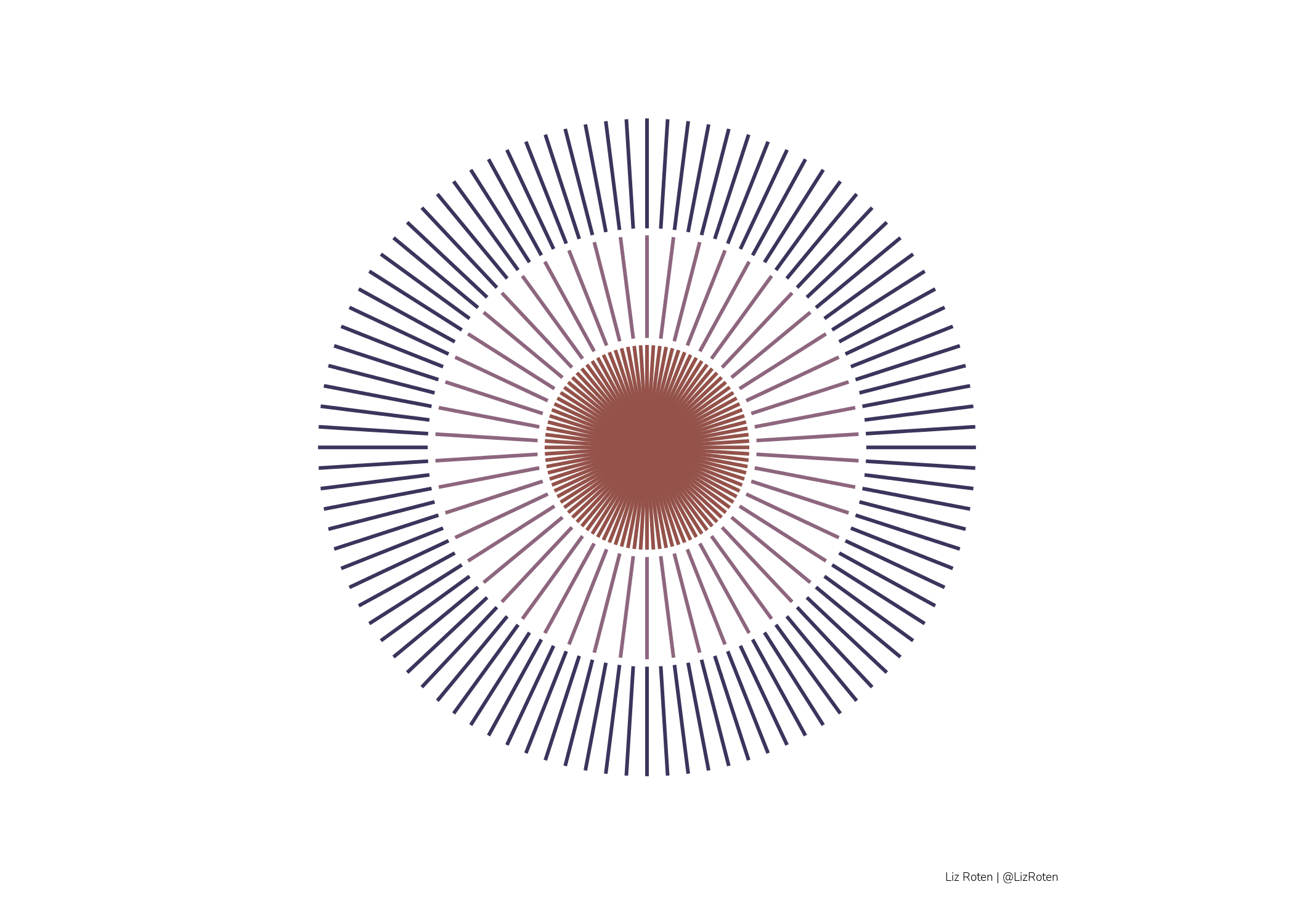increment = .05

circle_1 = tibble(
x = seq(from = 0, to = 5, by = increment),
xend = seq(from = 0, to = 5, by = increment),
y = 0,
yend = 1.5,
type = LETTERS)

circle_2 = tibble(
x = seq(from = 0, to = 5, by = increment),
xend = seq(from = 0, to = 5, by = increment),
y = 1.5,
yend = 3,
type = LETTERS)

circle_3 = tibble(
x = seq(from = 0, to = 5, by = increment ),
xend = seq(from = 0, to = 5, by = increment  ),
y = 3,
yend = 4.5,
type = LETTERS)

palette_values = c("#7A443E",
colorspace::lighten("#7A443E", 0.5),
colorspace::lighten("#7A443E", 0.8))

bind_rows(circle_1, circle_2, circle_3) %>%
ggplot(.) +
geom_segment(aes(x = x,
y = y,
xend = xend,
yend = yend,
color = type),
show.legend = F) +
scale_color_manual(values = palette_values) +
labs(caption = my_caption) +
coord_polar() +
theme_void() +
theme(
plot.caption = element_text(family = "Nunito Light",
color = "black",
hjust = 1,
size = 14),
plot.caption.position = "plot",
plot.margin = unit(c(.5, .5, .5, .5), "cm")
)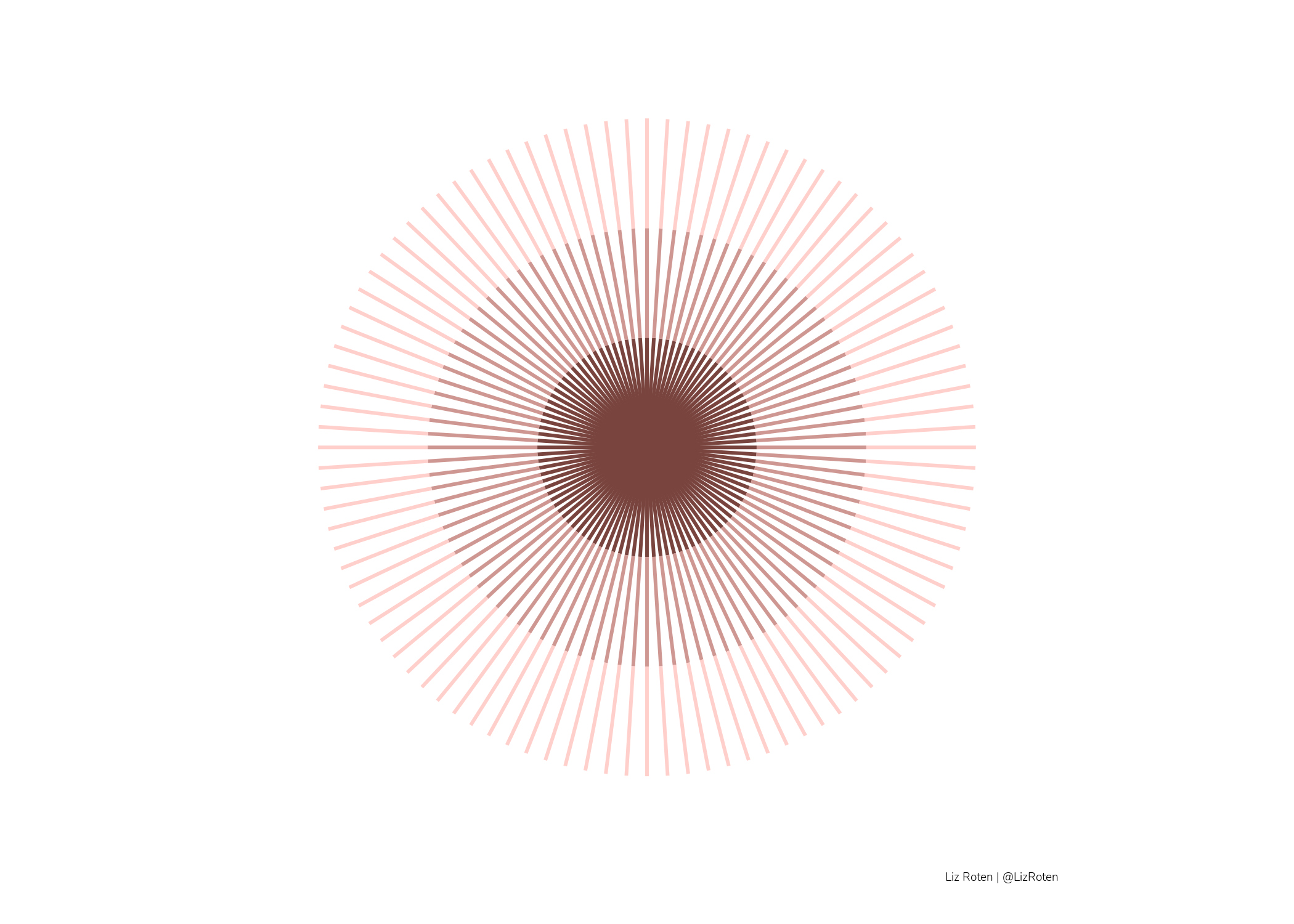## Florals

I fell in love with the opening slide in Ijeamaka Anyene’s talk at RLadies Johannesburg, so I wanted to give it a try!

set.seed(246153)

burst <- tibble(
x = seq(0, 10, by = 0.3),
xend = seq(0,10, by = 0.3),
y =  0,
yend = 0.4,
yend_jitt = jitter(yend, 2.6),
yend_jitt_point = yend_jitt

)

plot_burst <- ggplot() +
geom_segment(data = burst,
aes(x = x,
y = y,
xend = xend,
yend = yend_jitt),
color = "#F4AC32",
lwd = 0.5) +
geom_point(data = burst,
aes(x = x,
y = yend_jitt),
color = "#F4AC32",
size = 1.5
) +
coord_polar() +
theme_void()

bursts <- quote(expr = {cowplot::draw_plot(plot_burst,
scale = runif(1, min = 1.2, max = 3),
x = runif(1, min = 0, max = 10),
y = runif(1, min = 0, max = 5))})

plot_all <- cowplot::ggdraw(xlim = c(0,12),
ylim = c(0,6)) +
eval(bursts) +
eval(bursts) +
eval(bursts) +
eval(bursts) +
eval(bursts) +
eval(bursts) +
eval(bursts) +
eval(bursts) +
eval(bursts) +
labs(caption = my_caption) +
theme(plot.background = element_rect(fill = "#565254",
color = NA,
size = 0),
panel.background = element_rect(fill = "#565254",
color = NA,
size = 0),
plot.caption = element_text(family = "Nunito Light",
color = "white",
hjust = 1,
size = 14),
plot.caption.position = "plot",
plot.margin = unit(c(.5, .5, .5, .5), "cm")
)

plot_all